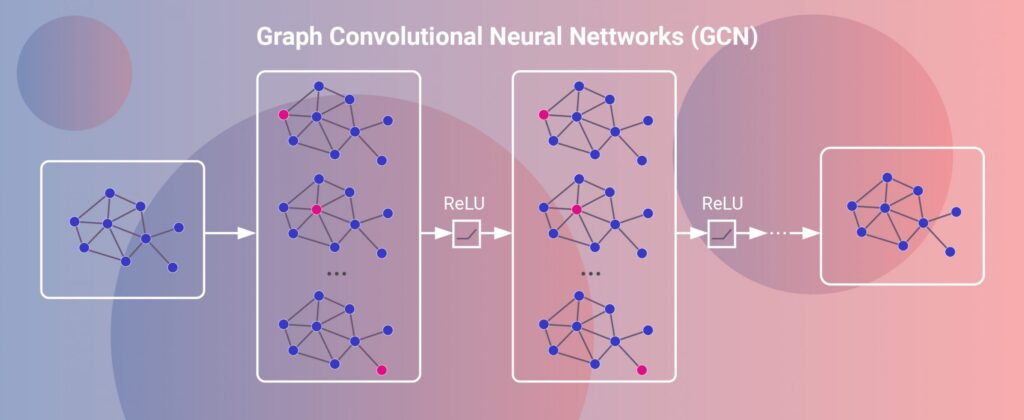## Testing 2

Similar Posts: None Found

## Route optimization of a pub crawl with ORS and

Similar Posts: None Found## Introduction

Introduction Introduction¶ Graph Neural Networks (GCNs) are an extension of the familiar Convolutional Neural Network to arbitrary topologies. Given the graph $G=\{V,E\}$, Gilmer et al. (2017) define the message-passing framework of GCNs as $$x_{i}^{l+1} = \Theta^{l}(n_{i}^{l},\gamma(x_{i}^{l},\{x_{j}^{l}:j\in{}N^{1}_{i}\},e_{ij}))$$ where $N_{i}^{1}$ is the 1-neighborhood of vertex $n_{i}$, and $l$ indexes the $l$-th layer of the model. Both $x_{i}^{l}\in{}R^{n}$ …